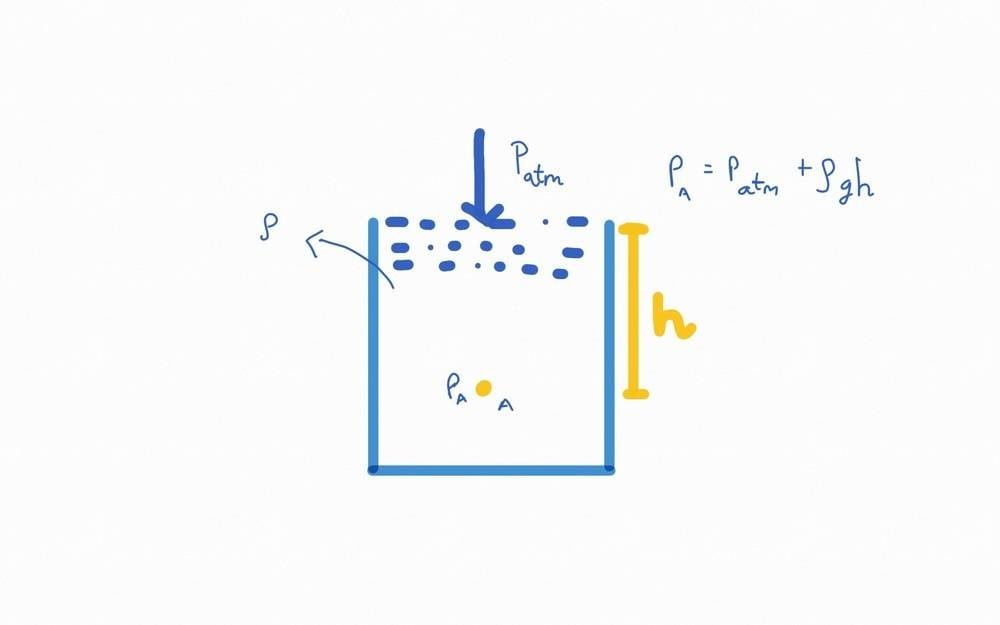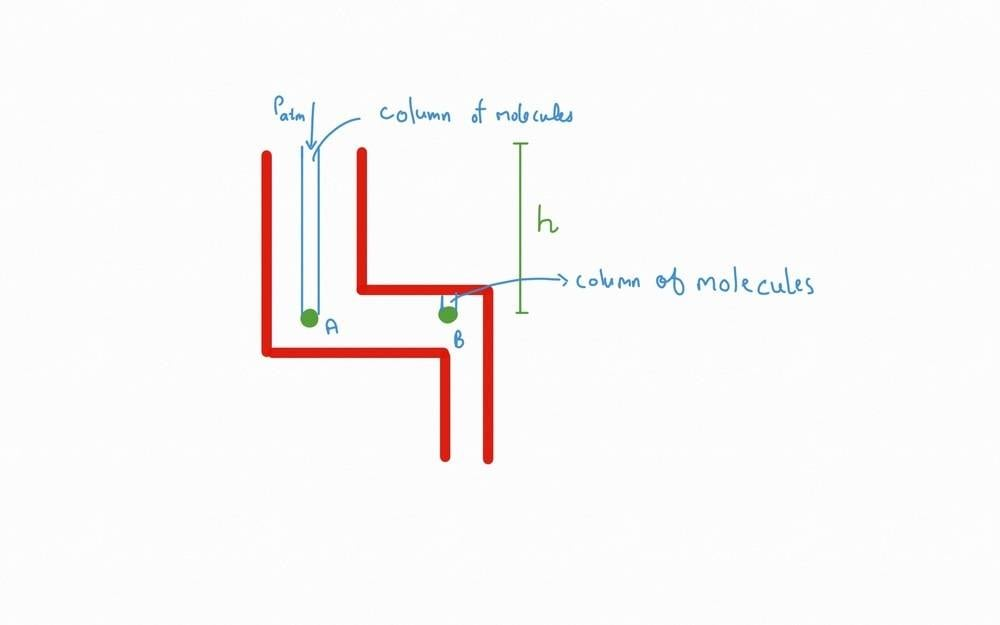# Pressure on a sample of fluid (at rest)

Question 1
Consider a sample at a height ##ℎ## below the interface of air and the fluid. The pressure on the sample is given by ##𝑃_𝑎=𝑃_{𝑎𝑡𝑚}+𝜌𝑔ℎ##.
My question is does 𝑃𝑎 include the pressure due to the particles that are to the side of the sample?Question 2
Now consider this setup.In this setup the two samples, say ##A## and ##B##, must experience same pressure i.e. ##P_a = P_b ## due to Pascals law. But the column of molecules above the sample is different. Isn't? Above B there are less number of molecules. On the other hand, above A there are more number of molecules and also ##P_{Atm}## adds to it. Due to this, the ##P_a > 𝑃_b##. But this isn't the case. When I asked this question to my teacher, he said that around the sample ##B## there are particles to the left that also exert pressure on it due to which ## P_a = P_b##
If this it true, then doesn't particles to the side of Sample ##A## also exert pressure on ##A##?
I asked the first question because of the second question.

Chestermiller
Mentor
Do you think that the pressure exerted downward by the horizontal pipe section above B is atmospheric pressure?

•Kaushik, dsaun777 and Dale
Do you think that the pressure exerted downward by the horizontal pipe section above B is atmospheric pressure?
No.
My understanding of pressure as of now is that as we go deeper into the ocean the column of water molecules vertically above that point increases. Hence, weight of those water molecules together is more than that of a point near the sea level. Hence, pressure at a point deeper will be more.

What is there in my mind is that,
##Weight## (of column of molecules vertically above the sample) ##\propto Pressure##

Last edited:
Chestermiller
Mentor
No.
My understanding of pressure as of now is that as we go deeper into the ocean the column of water molecules vertically above that point increases. Hence, weight of those water molecules together is more than that of a point near the sea level. Hence, pressure at a point deeper will be more.

What is there in my mind is that,
##Weight## (of column of molecules vertically above the sample) ##\propto Pressure##
Good. So now you understand why the pressure at B is the same as at A, right?

Good. So now you understand why the pressure at B is the same as at A, right?
No I still didn't get it. Is ##P_{wall}=P_{atm}##? If yes, then the pressure exerted by the column of water molecules on B is less. So as ##P_{Acolumn}## (column of water molecules above A) >##P_{Bcolumn}##(column of water molecules above B). Isn't? So this again bring a pressure difference between these two samples.

Chestermiller
Mentor
No I still didn't get it. Is ##P_{wall}=P_{atm}##? If yes, then the pressure exerted by the column of water molecules on B is less. So as ##P_{Acolumn}## (column of water molecules above A) >##P_{Bcolumn}##(column of water molecules above B). Isn't? So this again bring a pressure difference between these two samples.
No. The pressure at the wall above B is not atmospheric. According to Pascal's law, pressure is transmitted horizontally. That means that the pressure at B is the same as at A. Otherwise, fluid would be flowing from A to B. So the pressure at the wall above B is $$p_w=P_B-\rho g \delta=P_A-\rho g \delta=p_{atm}+\rho g (h-\delta)$$where ##\delta## is the vertical distance between point B and the wall above.

•Kaushik
Why is the pressure transmitted horizontally? Is it because of the Isotropicity of pressure?
Is it this horizontal pressure that makes ##P_b = P_a##?

Mister T
Gold Member
But the column of molecules above the sample is different. Isn't?

No,

Above B there are less number of molecules.

No, there aren't. Your confusion arises over the meaning of the word "above". In this context, any molecule with a height greater than some point in the fluid (for example, the place where you are measuring the pressure) is considered to be above that point. For example, the roof of my house is above me, even when I step outside of my house and walk across my lawn.

•Kaushik
No,

No, there aren't. Your confusion arises over the meaning of the word "above". In this context, any molecule with a height greater than some point in the fluid (for example, the place where you are measuring the pressure) is considered to be above that point. For example, the roof of my house is above me, even when I step outside of my house and walk across my lawn.
But in the context of pressure, how does the molecule above A affect Pressure exerted on B?

Mister T
Gold Member
But in the context of pressure, how does the molecule above A affect Pressure exerted on B?
The same way as it affects the pressure at A.

•Kaushik
The same way as it affects the pressure at A.
Is it possible to get a molecular explanation of why that is affecting the pressure exerted on B?

Chestermiller
Mentor
Why is the pressure transmitted horizontally? Is it because of the Isotropicity of pressure?
Is it this horizontal pressure that makes ##P_b = P_a##?
Yes and yes

•Kaushik
Mister T
Gold Member
Is it possible to get a molecular explanation of why that is affecting the pressure exerted on B?
Can you provide an explanation of why that is affecting the pressure exerted on A?

•Kaushik
Can you provide an explanation of why that is affecting the pressure exerted on A?
There are molecules above A and the weight of these molecules are the reason why pressure is exerted on A.

Mister T
Gold Member
There are molecules above A and the weight of these molecules are the reason why pressure is exerted on A.
Every molecule's that's above A is also above B.

•Kaushik
Every molecule's that's above A is also above B.
Please excuse me, but my question is how does it affect B? I understand that you mean the molecules above A is above B. But how does it exert pressure on B? I am not able to visualize it.
Thanks!

PS - Is the representation in the figure right? or Can you show how it affects B in the given diagram?

#### Attachments

Mister T
Gold Member
Please excuse me, but my question is how does it affect B?

You have already told us that you understand how it affects A. It affects B in the same way.

PS - Is the representation in the figure right?

I don't understand what the arrows in the figure represent.

•Kaushik
I don't understand what the arrows in the figure represent.
The arrow shows how the pressure is transmitted.
It affects B in the same way.
I said that the weight of the molecules above A (they are vertically above it) is the reason for the Pressure On A. But in the case of B, there are not as much molecules as there are vertically above A. So how can Weight of molecules above A affect the pressure on B.
Excuse Me if I am irritating you with my questions. Hope you understand!

Mister T
Gold Member
The arrow shows how the pressure is transmitted.

Then the figure is not correct. Pressure is not transmitted by arrows.

I said that the weight of the molecules above A (they are vertically above it) is the reason for the Pressure On A. But in the case of B, there are not as much molecules as there are vertically above A.

We seem to be going in circles here. I thought you had already agreed that everything above A is also above B.

•Kaushik
Then the figure is not correct. Pressure is not transmitted by arrows.

We seem to be going in circles here. I thought you had already agreed that everything above A is also above B.
Yes I did agree to your statement that everything above A is above B. But my question which is not yet answered is how does the weight of the molecule vertically above A affect the pressure on B? The pressure on A is affected by the molecules above it is self explanatory. But how does those molecules that are above A affect pressure on B?

For e.g. When there is a lot of load above my head the pressure on my head is due to the weight of the load. But it doesn't affect the person who is standing next to me in anyways.
This is what I am thinking of when I think of pressure. But it seems wrong in the context of pressure. This is the reason why I am asking how the weight of molecules (load) affect pressure on B (the person on whom there is no load).
Thanks! Hope you understand my question.

Dale
Mentor
2020 Award
I said that the weight of the molecules above A (they are vertically above it) is the reason for the Pressure On A.
This is a bad way to think of pressure. In hydrostatic equilibrium the weight of the molecules in a column above A gives the change in the pressure from the top of the column to the bottom of the column above A. In situations besides hydrostatic equilibrium the weight is largely irrelevant to the pressure, but even when it is relevant it gives the change in pressure, not the pressure itself.

•Kaushik
hutchphd
Homework Helper
Thanks! Hope you understand my question.
1. Pressure in a still fluid is exerted isotropically.
2. The upward pressure at point A must be sufficient to hold up that column (otherwise it would flow)
3. The pressure at B must be equal to A because there are no intervening forces (walls, gravity,etc)
Maybe that helps....

•Kaushik
Mister T
Gold Member
The pressure on A is affected by the molecules above it is self explanatory.
Not to me it isn't. The fact that you think it self-explanatory is at the heart of your confusion.

•Kaushik
jbriggs444
Homework Helper
Let us back up a bit and try to explain the self-explanatory.

Consider a small cube-shaped volume of water whose top is at depth ##h## below the surface. What the the pressure on its top surface?

For convenience, let us use "##l##" for the length of the sides, "##a##" for the area of the faces and "##v##" to denote its volume. The density of the water is ##\rho## and the acceleration of gravity is ##g##.

The top surface supports a vertical column of water that extends up to the surface. The volume of that column is given by ##ah##. Its weight is given by ##\rho g a h##. The pressure on the top surface is force divided by area: ##\frac{\rho g a h}{a}= \rho g h##

Which is exactly as expected, of course.

What is the pressure on the bottom surface of the cube? We can skip the formulas. The same reasoning applies. The pressure on the bottom surface is just a little higher than the pressure on the top surface because the bottom surface is just a little deeper.

No surprises there.

What is the pressure on the sides of the cube?

If that pressure were zero, what would you expect to happen to the water in the cube? It would get squished out the sides. But when the water is at equilibrium, that is not happening. So if the water is at equilibrium (not flowing, not sloshing, not accelerating), what must the pressure on the sides be?

••Vanadium 50, russ_watters, hutchphd and 1 other person
So if the water is at equilibrium (not flowing, not sloshing, not accelerating), what must the pressure on the sides be?
It must be equal to the pressure on the top surface so that the fluid doesn't flow?